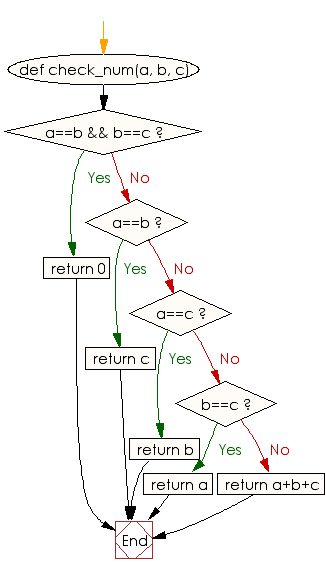﻿ Ruby Basic exercises: Check three given integers and return their sum - w3resource# Ruby Basic Exercises: Check three given integers and return their sum

## Ruby Basic: Exercise-52 with Solution

Write a Ruby program to check three given integers and return their sum. However, If one of the values is the same as another of the values, it does not count towards the sum.

Ruby Code:

``````def check_num(a, b, c)
if a==b && b==c
return 0
elsif a==b
return c
elsif a==c
return b
elsif b==c
return a
else
return a+b+c
end
end
print check_num(5, 5, 5),"\n"
print check_num(5, 5, 7),"\n"
print check_num(5, 7, 5),"\n"
print check_num(7, 5, 5),"\n"
print check_num(1, 2, 3),"\n"
``````

Output:

```0
7
7
7
6
```

Flowchart:Ruby Code Editor: